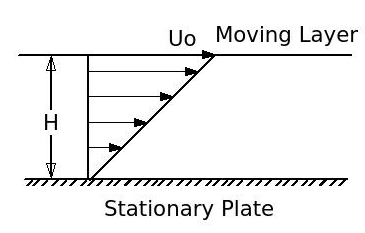# Fluid Flow and Heat Transfer - 1 - MCQs with Answers

## Fluid Flow and Heat Transfer – 1 – MCQs with Answers

1. The fluid flow in which the fluid particles in one layer do not mix with the fluid particles in the other layer is called as

a. laminar flow
b. turbulent flow
c. layer flow
d. none of the above

2. Generally, all the fluid particles in flowing fluid

a. flow at a constant velocity
b. flow at various velocities
c. flow at a velocity as high as possible
d. none of the above

ANSWER: b. flow at various velocities

3. What is the relation between the rate of convection heat transfer and the rate of mixing in turbulent fluid flow?

a. the rate of convection heat transfer decreases with increase in the rate of mixing in turbulent fluid flow
b. the rate of convection heat transfer increases with increase in the rate of mixing in turbulent fluid flow
c. the rate of convection heat transfer does not affected by the change in the rate of mixing in turbulent fluid flow
d. none of the above

ANSWER: b. the rate of convection heat transfer increases with increase in the rate of mixing in turbulent fluid flow

4. Viscosity of a fluid can be defined as

a. change in density of the fluid per unit temperature
b. flow resistance offered by the fluid
c. flow velocity change
d. none of the above

ANSWER: b. flow resistance offered by the fluid

5. Which of the following fluid can be considered as an ideal fluid?

a. viscous fluid
b. non-viscous fluid
c. compressible fluid
d. all of the above

6. Consider a fluid of height H, flowing on a stationary plate as shown in figure. The top most layer of the fluid is moving with velocity U0. Which of the following condition is correct for the given condition?a. The shear stress (τ) is directly proportional to (H x U0)
b. The shear stress (τ) is directly proportional to (H / U0)
c. The shear stress (τ) is directly proportional to (U0 / H)
d. none of the above